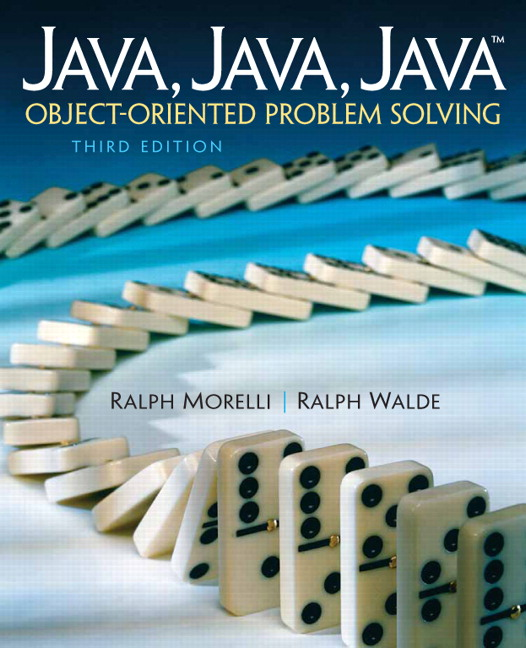# Java, Java, Java: Object-Oriented Problem Solving, 2022E

## Section9.3Simple Array Examples

The program in Listing 9.3.1 creates two arrays of ten elements each and displays their values on the Java console.
In this example, the elements of intArr have not been given initial values whereas the elements of realArr have been initialized. Note the use of the integer constant ARRSIZE to store the arrays’ size. By using the constant in this way, we do not have to use the literal value 10 anywhere in the program, thereby making it easier to read and to modify the program. If we want to change the size of the array that the program handles, we can just change the value of ARRSIZE. This is an example of the maintainability principle.
Note the use of the static qualifier throughout the PrintArrays class. This enables us to refer to the array and the other variables from within the main() method. If intArr were not declared static, we would get the compiler error attempt to make static use of a non-static variable.
This use of static is justified mainly as a coding convenience rather than a principle of object-oriented design. The only examples we’ve seen so far in which static elements were a necessary design element were the use of static elements in the Math class —Math.PI and Math.sqrt() — and the use of static final variables in TwoPlayerGame — e.g., TwoPlayerGame.PLAYER_ONE.
For large arrays, it is not always feasible to initialize them in an initializer statement. Consider the problem of initializing an array with the squares of the first 100 integers. Not only would it be tedious to set these values in an initializer statement, it would also be error prone, since it is relatively easy to type in the wrong value for one or more of the squares.
The example in Listing 9.3.5 creates an array of 50 integers and then fills the elements with the values 1, 4, 9, 16, and so on. It then prints the entire array.
This example illustrates some important points about the use of array variables. The array’s elements are individual storage locations. In this example, intArr has 50 storage locations. Storing a value in one of these variables is done by an assignment statement:
intArr[k] = (k+1) * (k+1);

The use of the variable k in this assignment statement allows us to vary the location that is assigned on each iteration of the for loop. Note that in this example, k occurs as the array index on the left-hand side of this expression, while k+1 occurs on the right-hand side as the value to be squared.
The reason for this is that arrays are indexed starting at 0 but we want our table of squares to begin with the square of 1. So the square of some number n+1 will always be stored in the array whose index is one less than the number itself — that is, n.
An array’s length variable can always be used as a loop bound when iterating through all elements of the array:
for (int k = 0; k < intArr.length; k++)
intArr[k] = (k+1) * (k+1);

However, it is important to note that the last element in the array is always at location length-1. Attempting to refer to intArr[length] would cause an IndexOutOfBoundsException because no such element exists.

### ExercisesSelf-Study Exercise

#### 1.Square Roots.

Create an array of doubles and populate it with the square roots of the first 20 integers $$\geq$$ 1. [Use Math.sqrt(double).] Then write a loop to display the arrays elements.